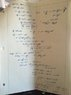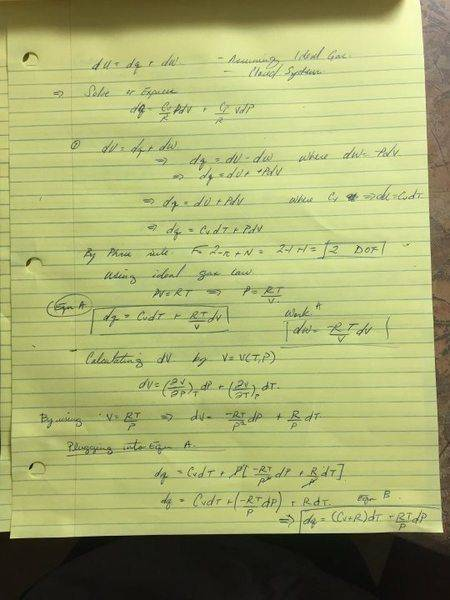# Rearrangement of the First Law of Thermodynamics

• HethensEnd25
HethensEnd25
Please post this type of questions in HW section using the template for showing your work.
For class we have been asked to show how the first law of thermodynamics

dU=dQ+dW

can be shown to be

dQ=(CV/R)*VdP +(Cp/R)*PdV

I have an answer, but am hesitant to say it is a final answer.

I will admit that while doing the problem I had trouble following what I was doing. Hence my posting the question. It seemed to me that because we know how state functions act and change with certain processes that much of the problem is a more of a "plug and chug" approach.

But I am concerned with the partial derivatives when rearranging this equation. How do certain parts cancel out or how does one approach the problem without them? Is it safe to assume that since you know how the state variables will act in a certain process that you can use that knowledge to provide a better or more exact answer to the problem?

Attached you will find a picture of the problem that I have done to the best of my ability (which is limited I must say)

If anyone can provide an explanation as to why certain things are done the way they are or correct my dissection of this problem I would be grateful for your time in the matter.

Best Regards,

D

#### Attachments

•IMG_1303.JPG
33.9 KB · Views: 580

HethensEnd25
assuming a closed system!**

Mentor
I'm working on it, but I haven't been able to solve it yet. Let's see what you've done so far.

Mentor
Correct me if I'm wrong but, if there is an R in the equation, this analysis must be specific to an ideal gas.

HethensEnd25
Yes you are correct. The assumption is that it is an ideal gas and a closed system.

attached find my answer thus far for the problem. I am still working out the last step holding T as a constant T=T(V,P)Mentor
This equation can not be applied in general because it can't describe the amount of heat transferred to an incompressible solid or liquid at constant pressure. For these cases, it would predict that dQ=0 (which is not necessarily correct). If it is not generic, it also cannot describe real gases beyond the ideal gas region. Therefore, the equation must apply exclusively to an ideal gas. The presence of the ideal gas constant R in the equation is a dead giveaway.

For a reversible path, $$dQ=TdS=C_vdT+PdV$$But, for an ideal gas, $$dT=\frac{PdV+VdP}{R}$$Therefore, $$dQ==C_v\frac{PdV+VdP}{R}+PdV=\frac{C_v}{R}VdP+\frac{(C_v+R)}{R}PdV$$But, for an ideal gas, $$C_v+R=C_p$$Therefore, $$dQ=\frac{C_v}{R}VdP+\frac{C_p}{R}PdV$$This equation applies only to an ideal gas and a reversible path.

HethensEnd25
dQ==Cv(PdV+VdP)/R+PdV=(Cv/R)*VdP+((Cv+R)/R)*PdV

for this part in particular how are you rearranging the terms of the ideal gas equation to get the end result algebraically?

I am most curious about the Cv+R that is obtained in the end result

I know that Cp=dU/dT +R=Cv+R

So would I simply just rearrange with T as a function of PV/R ?

I appreciate your clarification! I will continue to work the problem till I have the end result.

Best Regards,

D

Mentor
for this part in particular how are you rearranging the terms of the ideal gas equation to get the end result algebraically?
I'm just starting with the usual form of the first law and using the product rule to get dT in terms of dP and dV. The rest is easy.
I am most curious about the Cv+R that is obtained in the end result

I know that Cp=dU/dT +R=Cv+R
Yes. This is the substitution I made to get the final equation
So would I simply just rearrange with T as a function of PV/R ?
Yes. The idea is to eliminate dT by expressing dT in terms of dP and dV

•HethensEnd25
HethensEnd25
Thank you sir for the affirmation! My question has been answered for this particular problem.

It has been much appreciated.